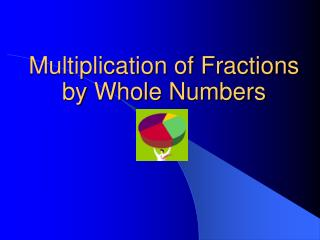DownloadDownload PresentationMultiplication of Fractions by Whole Numbers

# Multiplication of Fractions by Whole Numbers

Download Presentation## Multiplication of Fractions by Whole Numbers

- - - - - - - - - - - - - - - - - - - - - - - - - - - E N D - - - - - - - - - - - - - - - - - - - - - - - - - - -
##### Presentation Transcript

1. Multiplication of Fractions by Whole Numbers

2. Occasions You Would Multiply a Fraction by a Whole Number • You walk a 4 mile route halfway. ½ of 4 or ½ x 4 • You walk a ¾ mile route twice. 2 x ¾

3. Occasions You Would Multiply a Fraction by a Whole Number • A \$12 ticket to the magic show is on sale for 2/3 of the original price. 2/3 of \$12 or 2/3 x 12

4. How to Find the Fraction of a Whole Number • The first thing to remember is “of” means multiply in mathematics. of x =

5. How to Find the Fraction of a Whole Number • Step 1 - When you see a fraction problem you know when you read “of” in the problem you multiply. 1 x of 46 2

6. How to Find the Fraction of a Whole Number • Step 2 – Change your whole number to a fraction by adding a one underneath. 1 x 46 2 1

7. How to Find the Fraction of a Whole Number • Step 3 – Multiply your numerators straight across. 1 x 46 46 = 2 1

8. How to Find the Fraction of a Whole Number • Step 4 – Then multiply your denominators straight across. 1 x 46 46 = 2 1 2

9. How to Find the Fraction of a Whole Number • Step 5 – Simplify your answer. In this case we change an improper fraction to a mixed number or a whole number. 23 1 x 46 46 2) = 46 2 1 2

10. Multiply and Simplify 12 1 36 36 of x = 3) 36 3 1 3

11. Multiply and Simplify 3 1 18 18 of x = 6) 18 6 1 6

12. Multiply and Simplify 3 3 9 27 3 8 of x = 8) 8 1 8 27 24 3

13. Multiply and Simplify 2 7 6 42 5 8 of x = 8) 8 1 8 42 40 2 2 1 ÷ = 5 5 2 8 ÷ 2 4

14. Multiply and Simplify You’re really getting this! 4 1 12 12 1 8 of x = 8) 8 1 8 12 8 4 4 1 ÷ = 1 1 4 8 4 2 ÷